# How to delete attributes or rows of the exampleset automatically

Member Posts: 8Contributor II
in Help
Hi guys,
i am a new member of the Rapidminer community and would like know, how can i just remove or delete automatically several attributes or rows, which contain certain  kind values? For my apllication i dont need the time stamp and would like to delete them from my example set. Thank you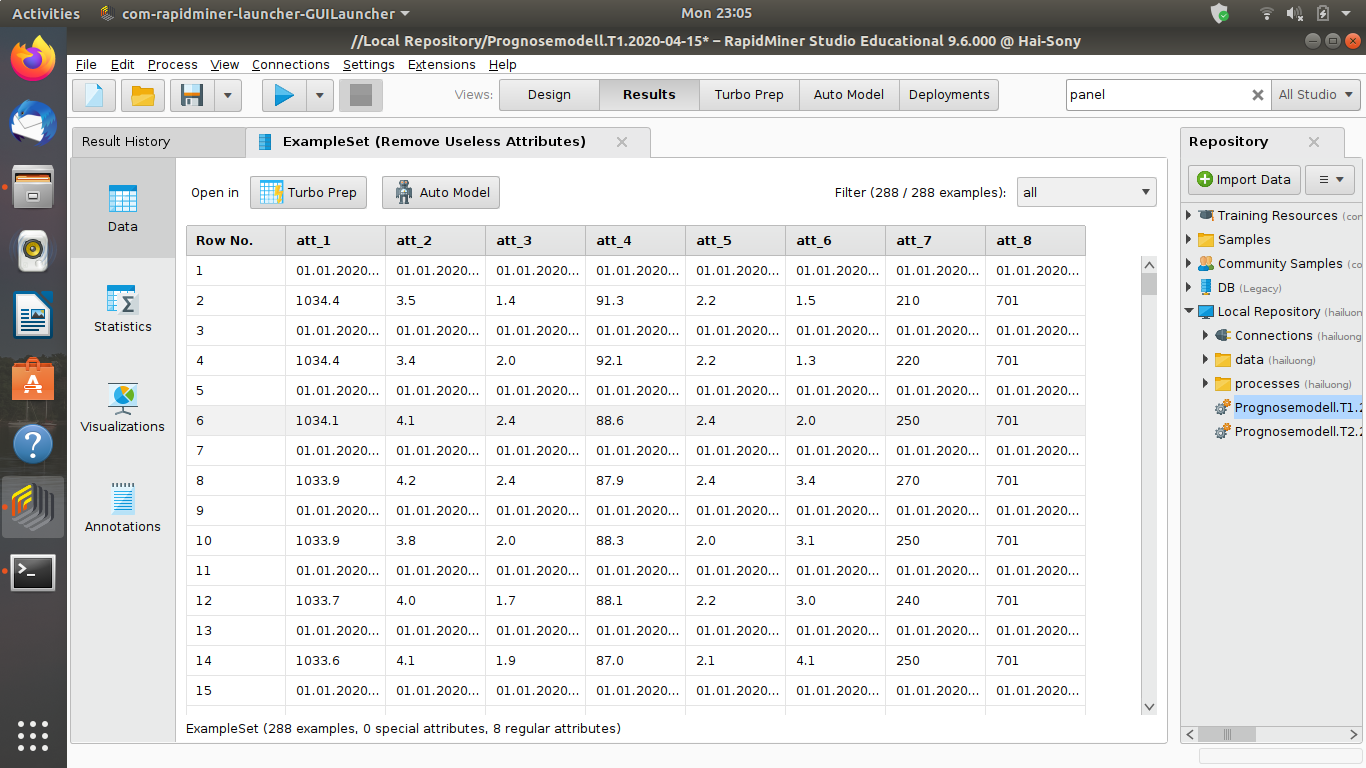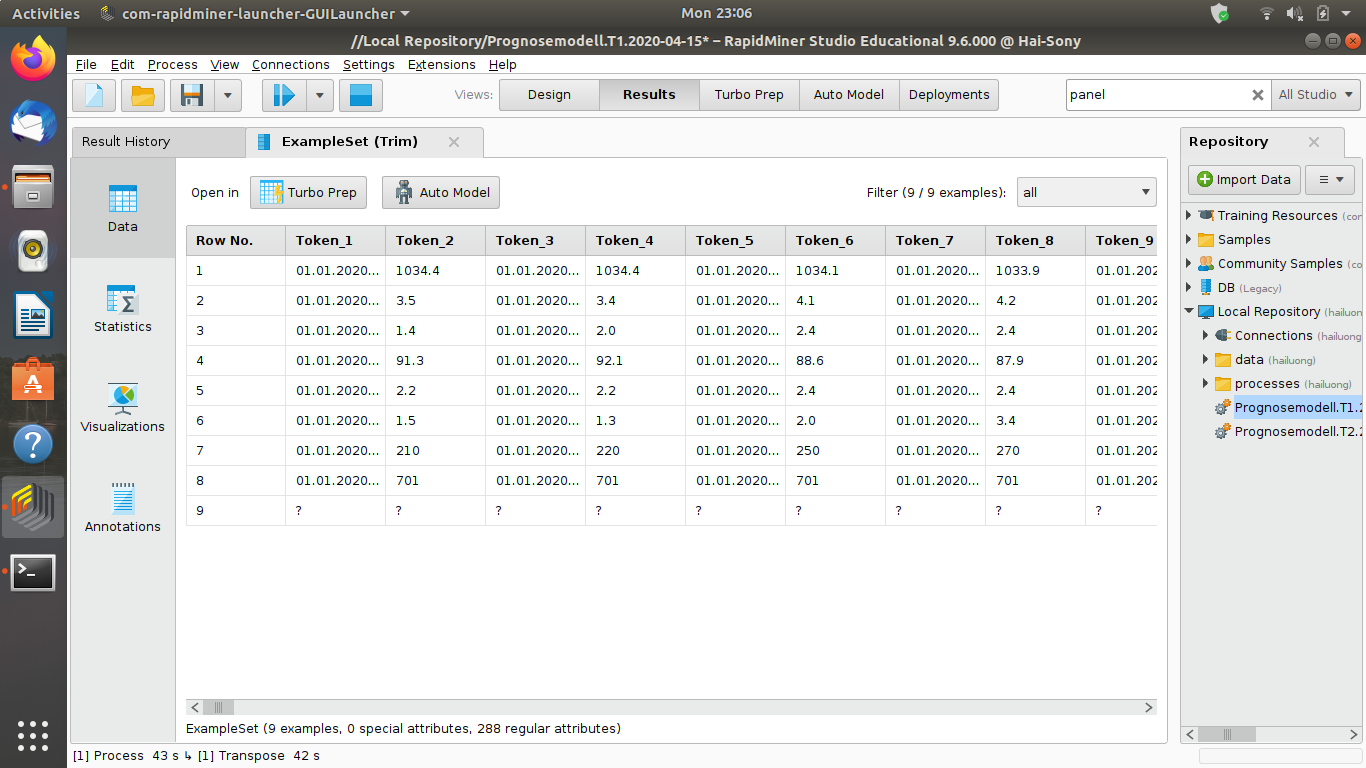• Moderator, RapidMiner Certified Analyst, Member Posts: 1,195Unicorn
edited May 2020
Hi @sharki,

I have maybe an idea. Can you share your dataset ?
In addition, can you elaborate :

i dont need the time stamp and would like to delete them from my example set

What do you want to do exactly ?

- if an attribute contains at least a date, you remove this attribute ?
- if a row contains at least a date, you remove the row ?

Regards,

Lionel
• Member Posts: 8Contributor II
edited May 2020
Hi @lionelderkrikor,thank you for your interest. My intention is, that i would like to extract the data of a application through its URL-Adresses. With the data i would like to create a exempleset, which should look like this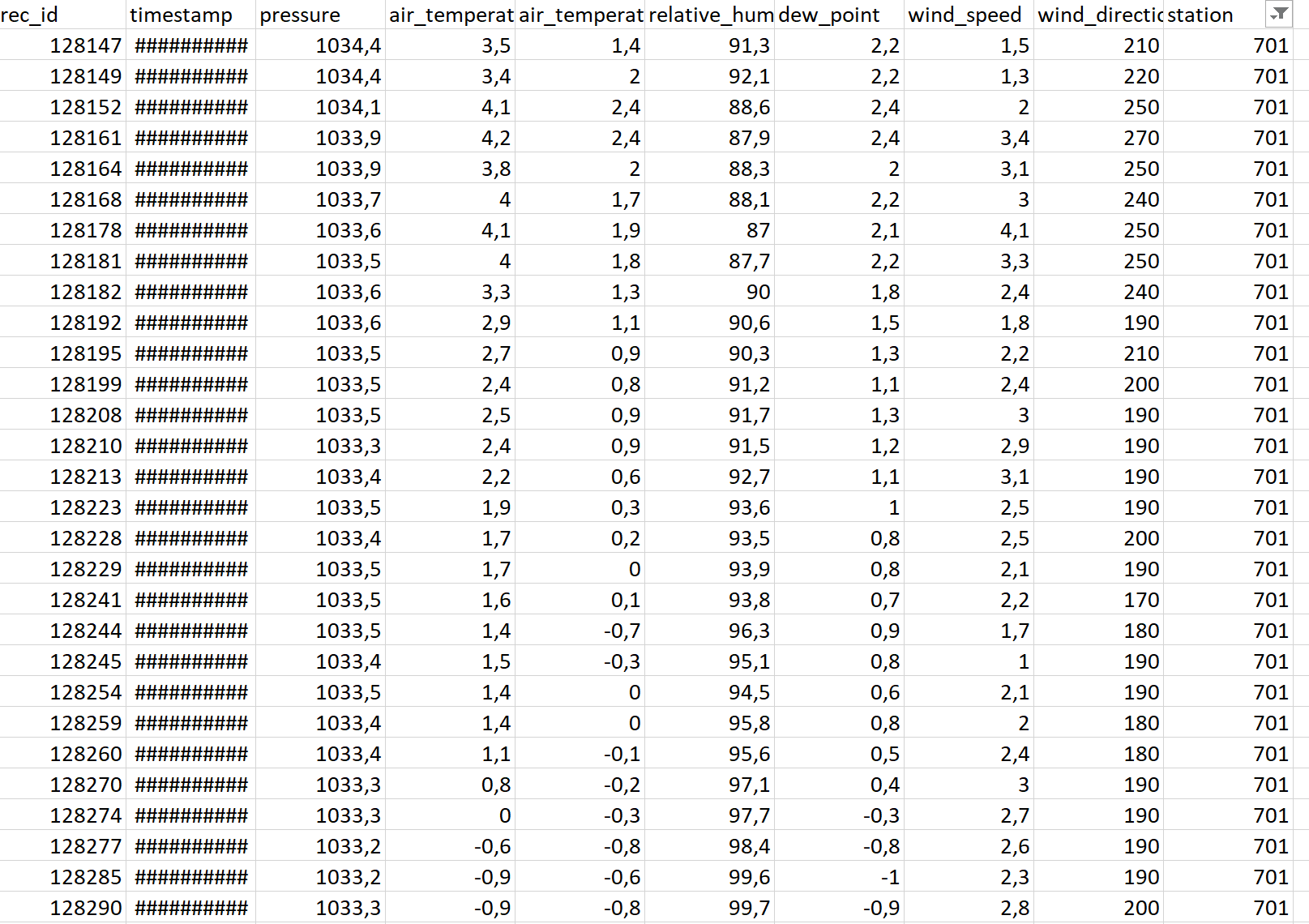Here is my process so far.

<?xml version="1.0" encoding="UTF-8"?>

-<process version="9.6.000">

-<context>

<input/>

<output/>

<macros/>

</context>

-<operator name="Process" expanded="true" compatibility="9.6.000" class="process" activated="true">

<parameter value="init" key="logverbosity"/>

<parameter value="2001" key="random_seed"/>

<parameter value="never" key="send_mail"/>

<parameter value="30" key="process_duration_for_mail"/>

<parameter value="SYSTEM" key="encoding"/>

-<process expanded="true">

<parameter value="sheet number" key="sheet_selection"/>

<parameter value="1" key="sheet_number"/>

<parameter value="A1" key="imported_cell_range"/>

<parameter value="SYSTEM" key="encoding"/>

<parameter value="true" key="first_row_as_names"/>

<list key="annotations"/>

<parameter value="" key="date_format"/>

<parameter value="SYSTEM" key="time_zone"/>

<parameter value="English (United States)" key="locale"/>

-<list key="data_set_meta_data_information">

</list>

<parameter value="double_array" key="datamanagement"/>

<parameter value="auto" key="data_management"/>

</operator>

-<operator name="Get Pages" expanded="true" compatibility="9.3.001" class="web:retrieve_webpages" activated="true" y="34" x="179" width="90" height="68">

<parameter value="false" key="random_user_agent"/>

<parameter value="10000" key="connection_timeout"/>

<parameter value="true" key="follow_redirects"/>

<parameter value="GET" key="request_method"/>

<parameter value="none" key="delay"/>

<parameter value="1000" key="delay_amount"/>

<parameter value="0" key="min_delay_amount"/>

<parameter value="1000" key="max_delay_amount"/>

</operator>

-<operator name="Data to Documents" expanded="true" compatibility="9.3.001" class="text:data_to_documents" activated="true" y="34" x="313" width="90" height="68">

<parameter value="false" key="select_attributes_and_weights"/>

<list key="specify_weights"/>

</operator>

<operator name="Combine Documents" expanded="true" compatibility="9.3.001" class="text:combine_documents" activated="true" y="34" x="447" width="90" height="82"/>

-<operator name="Remove Document Parts" expanded="true" compatibility="9.3.001" class="text:remove_document_parts" activated="true" y="34" x="581" width="90" height="68">

<parameter value="item_time|item_value|" key="deletion_regex"/>

</operator>

-<operator name="Split Document into Collection" expanded="true" compatibility="2.4.000" class="operator_toolbox:split_document_into_collection" activated="true" y="187" x="45" width="90" height="82">

<parameter value="\n" key="split_string"/>

</operator>

-<operator name="Split" expanded="true" compatibility="9.6.000" class="split" activated="true" y="187" x="179" width="90" height="82">

<parameter value="value_type" key="attribute_filter_type"/>

<parameter value="Token" key="attribute"/>

<parameter value="" key="attributes"/>

<parameter value="false" key="use_except_expression"/>

<parameter value="nominal" key="value_type"/>

<parameter value="false" key="use_value_type_exception"/>

<parameter value="file_path" key="except_value_type"/>

<parameter value="single_value" key="block_type"/>

<parameter value="false" key="use_block_type_exception"/>

<parameter value="single_value" key="except_block_type"/>

<parameter value="false" key="invert_selection"/>

<parameter value="false" key="include_special_attributes"/>

<parameter value="," key="split_pattern"/>

<parameter value="ordered_split" key="split_mode"/>

</operator>

-<operator name="Replace" expanded="true" compatibility="9.6.000" class="replace" activated="true" y="187" x="313" width="90" height="82">

<parameter value="all" key="attribute_filter_type"/>

<parameter value="" key="attribute"/>

<parameter value="" key="attributes"/>

<parameter value="false" key="use_except_expression"/>

<parameter value="nominal" key="value_type"/>

<parameter value="false" key="use_value_type_exception"/>

<parameter value="file_path" key="except_value_type"/>

<parameter value="single_value" key="block_type"/>

<parameter value="false" key="use_block_type_exception"/>

<parameter value="single_value" key="except_block_type"/>

<parameter value="false" key="invert_selection"/>

<parameter value="false" key="include_special_attributes"/>

<parameter value="[-!"#\$%&'()*+/;:<=>?@\[\\\]_`{|}~]" key="replace_what"/>

<parameter value=" " key="replace_by"/>

</operator>

-<operator name="Trim" expanded="true" compatibility="9.6.000" class="trim" activated="true" y="187" x="447" width="90" height="82">

<parameter value="all" key="attribute_filter_type"/>

<parameter value="" key="attribute"/>

<parameter value="" key="attributes"/>

<parameter value="false" key="use_except_expression"/>

<parameter value="nominal" key="value_type"/>

<parameter value="false" key="use_value_type_exception"/>

<parameter value="file_path" key="except_value_type"/>

<parameter value="single_value" key="block_type"/>

<parameter value="false" key="use_block_type_exception"/>

<parameter value="single_value" key="except_block_type"/>

<parameter value="false" key="invert_selection"/>

<parameter value="false" key="include_special_attributes"/>

</operator>

<operator name="Transpose" expanded="true" compatibility="9.6.000" class="transpose" activated="true" y="187" x="581" width="90" height="82"/>

-<operator name="Select Attributes" expanded="true" compatibility="9.6.000" class="select_attributes" activated="true" y="340" x="447" width="90" height="82">

<parameter value="single" key="attribute_filter_type"/>

<parameter value="id" key="attribute"/>

<parameter value="" key="attributes"/>

<parameter value="false" key="use_except_expression"/>

<parameter value="attribute_value" key="value_type"/>

<parameter value="false" key="use_value_type_exception"/>

<parameter value="time" key="except_value_type"/>

<parameter value="attribute_block" key="block_type"/>

<parameter value="false" key="use_block_type_exception"/>

<parameter value="value_matrix_row_start" key="except_block_type"/>

<parameter value="true" key="invert_selection"/>

<parameter value="true" key="include_special_attributes"/>

</operator>

-<operator name="Remove Useless Attributes" expanded="true" compatibility="9.6.000" class="remove_useless_attributes" activated="true" y="340" x="581" width="90" height="82">

<parameter value="0.0" key="numerical_min_deviation"/>

<parameter value="1.0" key="nominal_useless_above"/>

<parameter value="false" key="nominal_remove_id_like"/>

<parameter value="0.0" key="nominal_useless_below"/>

</operator>

<connect to_port="Example Set" to_op="Get Pages" from_port="output" from_op="Read Excel"/>

<connect to_port="example set" to_op="Data to Documents" from_port="Example Set" from_op="Get Pages"/>

<connect to_port="documents 1" to_op="Combine Documents" from_port="documents" from_op="Data to Documents"/>

<connect to_port="document" to_op="Remove Document Parts" from_port="document" from_op="Combine Documents"/>

<connect to_port="document" to_op="Split Document into Collection" from_port="document" from_op="Remove Document Parts"/>

<connect to_port="example set input" to_op="Split" from_port="example set" from_op="Split Document into Collection"/>

<connect to_port="example set input" to_op="Replace" from_port="example set output" from_op="Split"/>

<connect to_port="example set input" to_op="Trim" from_port="example set output" from_op="Replace"/>

<connect to_port="example set input" to_op="Transpose" from_port="example set output" from_op="Trim"/>

<connect to_port="example set input" to_op="Select Attributes" from_port="example set output" from_op="Transpose"/>

<connect to_port="example set input" to_op="Remove Useless Attributes" from_port="example set output" from_op="Select Attributes"/>

<connect to_port="result 1" from_port="example set output" from_op="Remove Useless Attributes"/>

<portSpacing spacing="0" port="source_input 1"/>

<portSpacing spacing="0" port="sink_result 1"/>

<portSpacing spacing="0" port="sink_result 2"/>

</process>

</operator>

</process>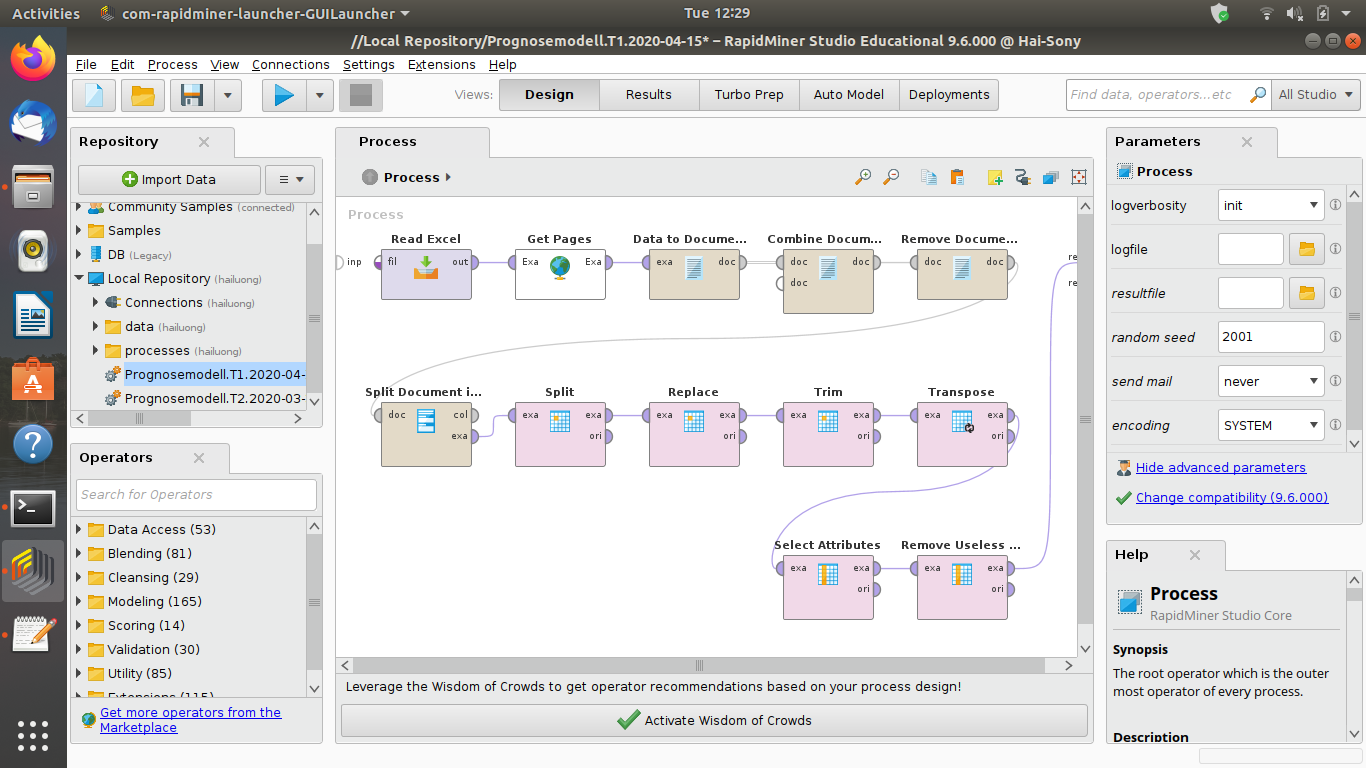So after Combine Documents Operator i got a Dataset, which look like that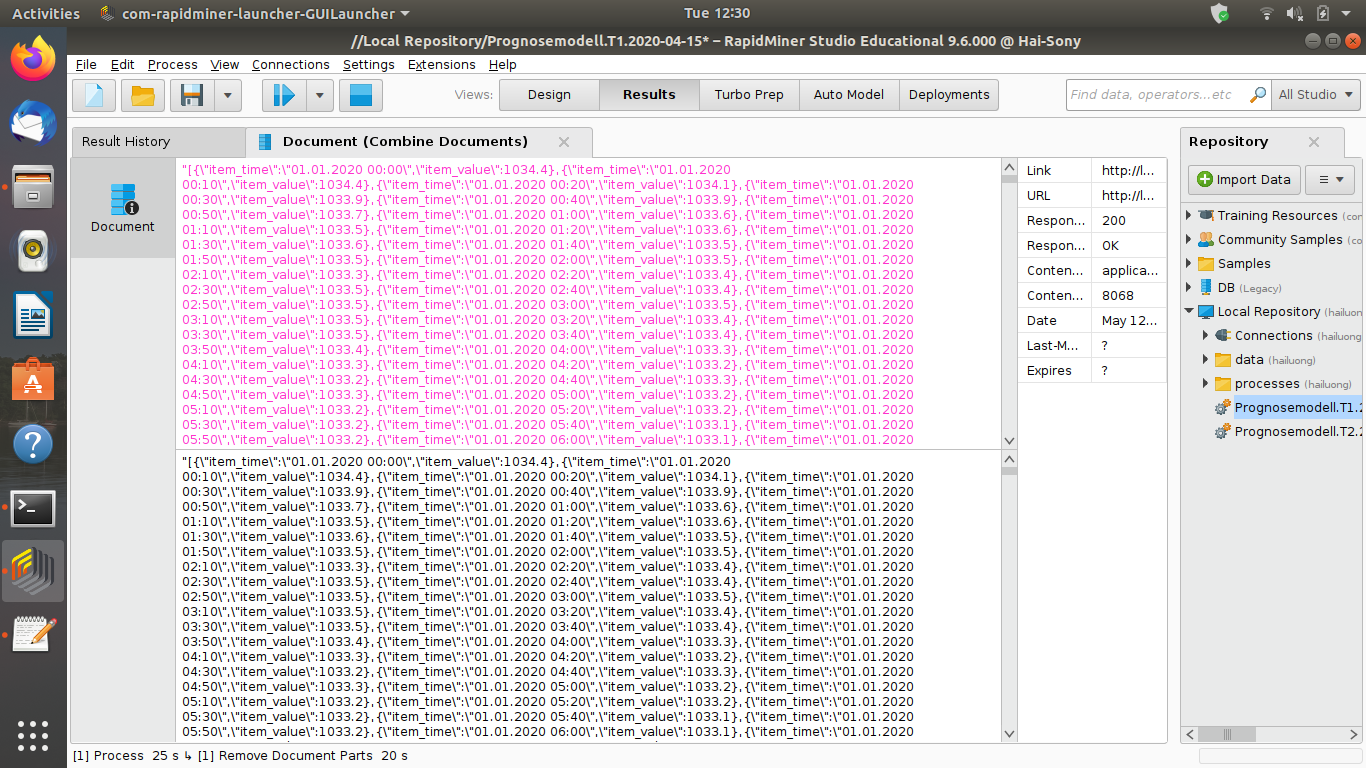the example set looks like that before transposeand at the end of the whole process like thatSo if you take a look at my first picture, then maybe you would know my intention of my set up. If i could clear up the time stamp in the rows, then i will get exact the same dataset like the one in the first picture. And because i work with a dynamic data set, therefore i would like to know to delete the rows, colums or unwanted values in my exampleset automatically, so that i would'nt just have to delete the rows, colums , attributes or values by hand. Sorry for the long answer. i hope, i could express well, what i would like to do.
• Moderator, RapidMiner Certified Analyst, Member Posts: 1,195Unicorn
Hi @sharki,

What is the complete pattern of your timestamp ? (ie DD.MM.YYYY ? or something else ..?)
In the screenshot you shared, the timestamp is truncated so, I can not determine it.

Regards,

Lionel
• Member Posts: 8Contributor II
Hi @lionelderkrikor

the pattern of the timestamp is DD.MM.YYYY HH:MM i guess. The application records every ten minutes different values of the parameters, which are measured by several sensores.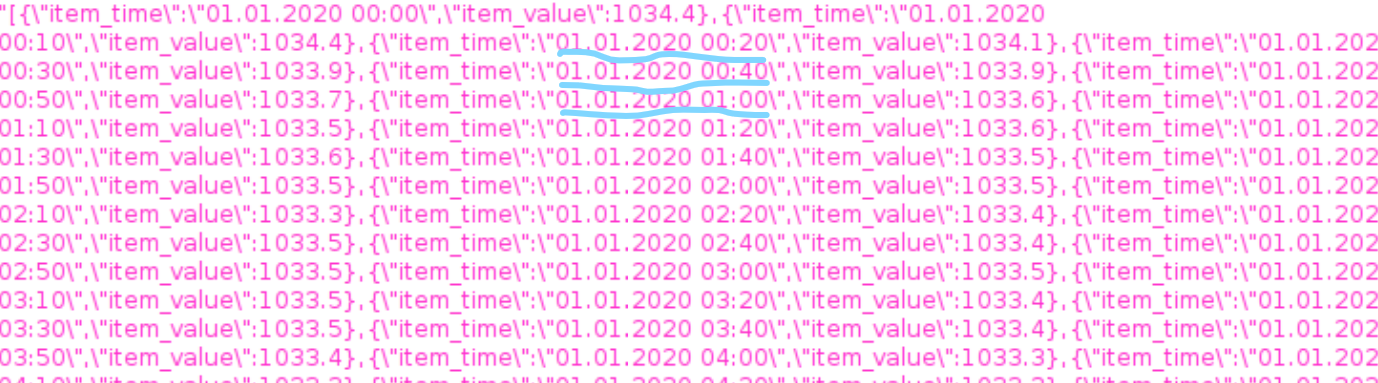• Member Posts: 8Contributor II
Hi @lionelderkrikor, you are just genius and a brillian  rapidminer magician! thanks for your effort! ^^
• Moderator, RapidMiner Certified Analyst, Member Posts: 1,195Unicorn
You're welcome, @sharki.

Good luck for your study !

Regards,

Lionel